Алгебра_9сынып_Геометриялық ықтималдылық_Қосымша 1
Оценка 4.6

# Алгебра_9сынып_Геометриялық ықтималдылық_Қосымша 1

Оценка 4.6
pdf
математика
13.05.2020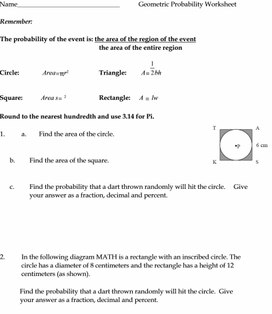Алгебра_9сынып_Геометриялық ықтималдылық_Қосымша 1.pdf

Name_______________________________           Geometric Probability Worksheet

Remember:

The probability of the event is: the area of the region of the event

the area of the entire region

Circle:              Area=πr2                  Triangle:         A=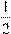bh

Square:           Area s= 2                    Rectangle:      A = lw

Round to the nearest hundredth and use 3.14 for Pi.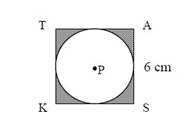1.                    a.        Find the area of the circle.

b.                  Find the area of the square.

c.                   Find the probability that a dart thrown randomly will hit the circle.     Give your answer as a fraction, decimal and percent.

2.                    In the following diagram MATH is a rectangle with an inscribed circle. The circle has a diameter of 8 centimeters and the rectangle has a height of 12 centimeters (as shown).

Find the probability that a dart thrown randomly will hit the circle.  Give your answer as a fraction, decimal and percent.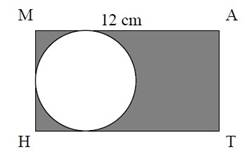3.                    In the following diagram, right triangle ABC is inscribed in a circle. It is given that AC = 26, BC = 24, AB=10 and AC is the diameter of the circle.

Find the probability that a dart thrown randomly will hit the triangle.  Give your answer as a fraction, decimal and percent.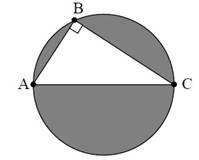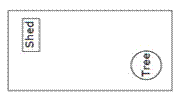Use the picture at the right for Questions 4 – 8.

4.                    A rectangular field measures 27 feet by 15 feet. Find the area of the field.

5.                    A small shed is on the field. Its dimensions are 8 feet by 10 feet. What is its area?

6.                    What is the probability that a single drop of rain that lands in the field would hit the shed? Give your answer as a fraction, decimal and percent.

7.                    What is the probability that a single drop of rain that lands in the field would not hit the shed? Give your answer as a fraction, decimal and percent.

8.                    CHALLENGE:  There is a large oak tree in one corner whose branches have a diameter of 20 feet. What is the probability that a single drop of rain that lands in the field would miss both the shed and the tree? (Assume the shed is not under the tree.)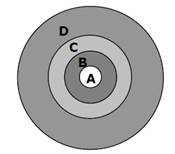Use the dartboard at the right for Questions 9 – 15.

A dartboard is made up of concentric circles with the following radii:

Circle A: r = 2 inches Circle B: r = 4 inches

Circle C: r = 6 inches

Circle D: r = 10 inches

9.                  Find the area of circle A.

10.              Find the area of circle B that is not covered by circle A

.

11.              Find the area circle C that is not covered by circle A or B.

12.              Find the area of the dartboard that is not covered by circles A, B, or C.

The circles on the dartboard are painted on a rectangular piece of corkboard that is 2 feet by 30 inches. Find the probability of each event, assuming the dart always lands on the corkboard.

13.              A random dart lands on circle B or C.

14.              A random dart lands just on circle B.

15.              A random dart will make a bull’s eye.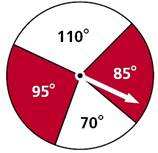Use the spinner for Questions 16 – 20.

16.              Find the probability that the spinner will land on the 95° region.

17.              Find the probability that the spinner will land on the 110° region.

18.              Find the probability that the spinner will land on the shaded region.

19.              Find the probability that the spinner will land on the non-shaded region.

20.              Find the probability that the spinner will land on a single region greater than 180°.

## What is the probability that a single drop of rain that lands in the field would not hit the shed?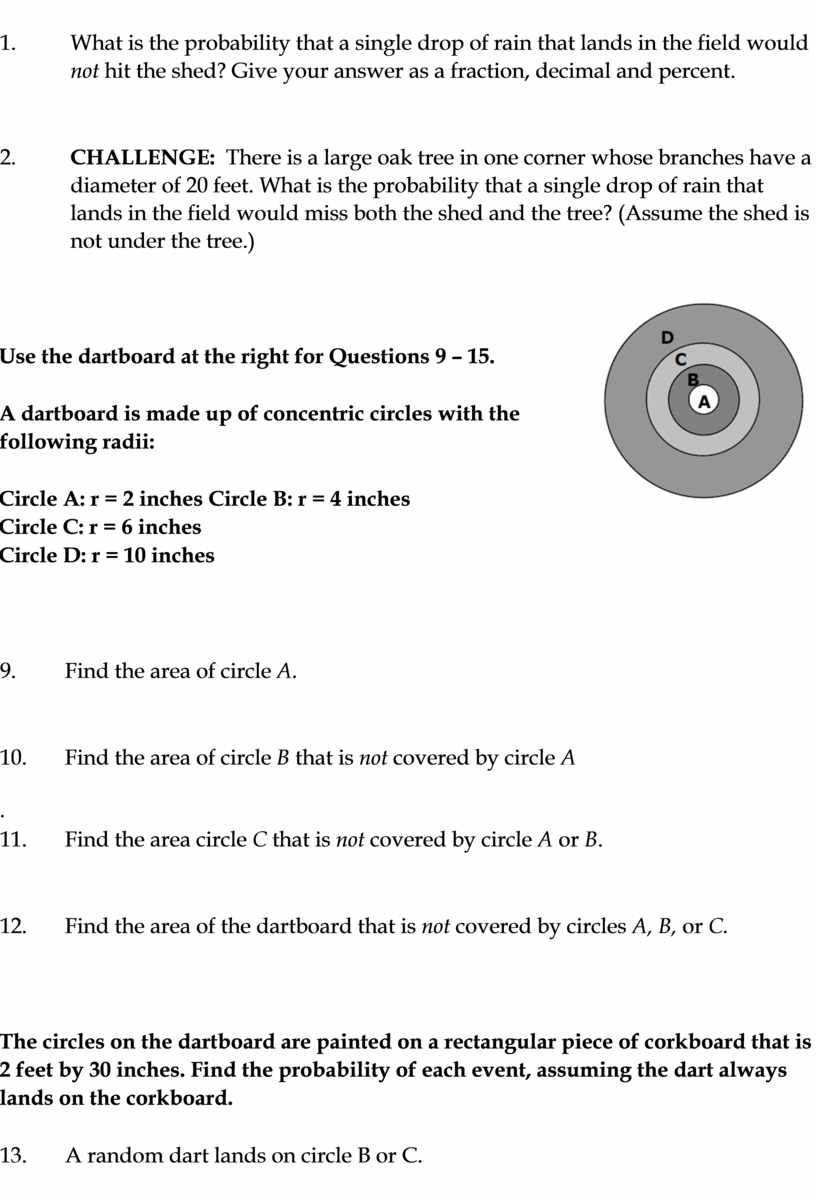Скачать файл